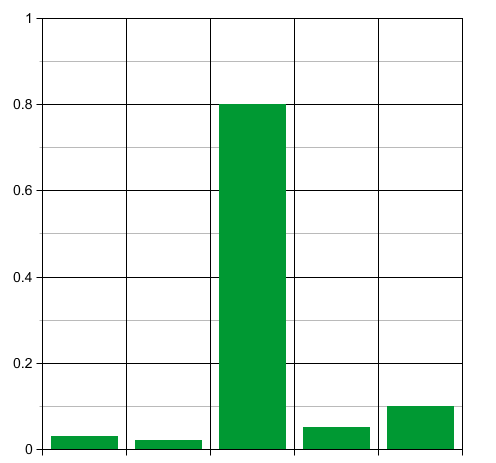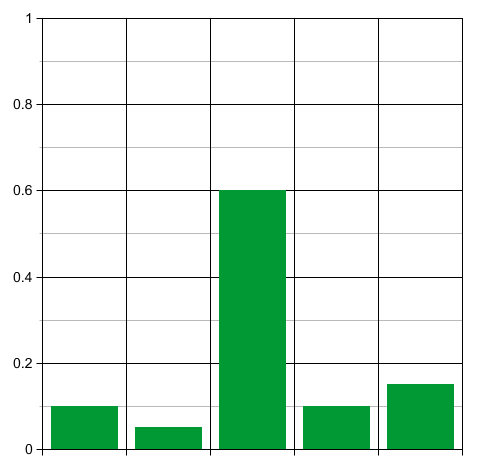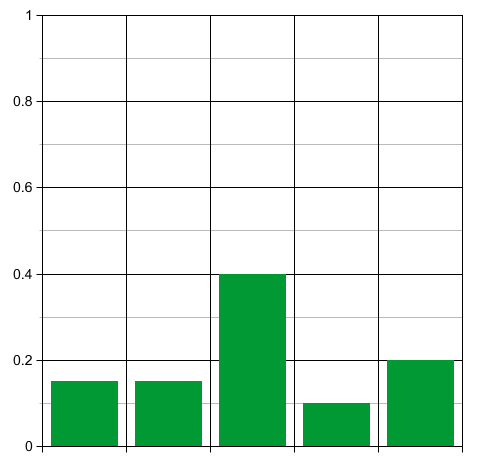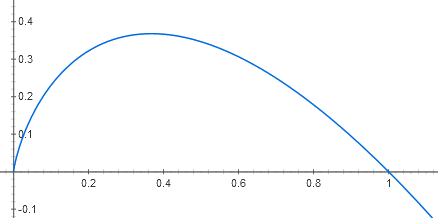## Information Geometry (Part 10)

#### John Baez

Last time I began explaining the tight relation between three concepts:

• entropy,
• information—or more precisely, lack of information,

and

• biodiversity.

The idea is to consider $n$ different species of 'replicators'. A replicator is any entity that can reproduce itself, like an organism, a gene, or a meme. A replicator can come in different kinds, and a 'species' is just our name for one of these kinds. If $P_i$ is the population of the $i$th species, we can interpret the fraction

$$\displaystyle{ p_i = \frac{P_i}{\sum_j P_j} }$$

as a probability: the probability that a randomly chosen replicator belongs to the $i$th species. This suggests that we define entropy just as we do in statistical mechanics:

$$\displaystyle{ S = - \sum_i p_i \ln(p_i) }$$

In the study of statistical inference, entropy is a measure of uncertainty, or lack of information. But now we can interpret it as a measure of biodiversity: it's zero when just one species is present, and small when a few species have much larger populations than all the rest, but gets big otherwise.

Our goal here is play these viewpoints off against each other. In short, we want to think of natural selection, and even biological evolution, as a process of statistical inference—or in simple terms, learning.

To do this, let's think about how entropy changes with time. Last time we introduced a simple model called the replicator equation:

$$\displaystyle{ \frac{d P_i}{d t} = f_i(P_1, \dots, P_n) \, P_i }$$

where each population grows at a rate proportional to some 'fitness functions' $f_i$. We can get some intuition by looking at the pathetically simple case where these functions are actually constants, so

$$\displaystyle{ \frac{d P_i}{d t} = f_i \, P_i }$$

The equation then becomes trivial to solve:

$$\displaystyle{ P_i(t) = e^{t f_i } P_i(0)}$$

Last time I showed that in this case, the entropy will eventually decrease. It will go to zero as $t \to +\infty$ whenever one species is fitter than all the rest and starts out with a nonzero population—since then this species will eventually take over.

But remember, the entropy of a probability distribution is its lack of information. So the decrease in entropy signals an increase in information. And last time I argued that this makes perfect sense. As the fittest species takes over and biodiversity drops, the population is acquiring information about its environment.

However, I never said the entropy is always decreasing, because that's false! Even in this pathetically simple case, entropy can increase.

Suppose we start with many replicators belonging to one very unfit species, and a few belonging to various more fit species. The probability distribution $p_i$ will start out sharply peaked, so the entropy will start out low:Now think about what happens when time passes. At first the unfit species will rapidly die off, while the population of the other species slowly grows:So the probability distribution will, for a while, become less sharply peaked. Thus, for a while, the entropy will increase!

This seems to conflict with our idea that the population's entropy should decrease as it acquires information about its environment. But in fact this phenomenon is familiar in the study of statistical inference. If you start out with strongly held false beliefs about a situation, the first effect of learning more is to become less certain about what's going on!

Get it? Say you start out by assigning a high probability to some wrong guess about a situation. The entropy of your probability distribution is low: you're quite certain about what's going on. But you're wrong. When you first start suspecting you're wrong, you become more uncertain about what's going on. Your probability distribution flattens out, and the entropy goes up.

So, sometimes learning involves a decrease in information—false information. There's nothing about the mathematical concept of information that says this information is true.

Given this, it's good to work out a formula for the rate of change of entropy, which will let us see more clearly when it goes down and when it goes up. To do this, first let's derive a completely general formula for the time derivative of the entropy of a probability distribution. Following Sir Isaac Newton, we'll use a dot to stand for a time derivative:

$$\begin{array}{ccl} \displaystyle{ \dot{S}} &=& \displaystyle{ - \frac{d}{dt} \sum_i p_i \ln (p_i)} \\ \\ &=& - \displaystyle{ \sum_i \dot{p}_i \ln (p_i) + \dot{p}_i } \end{array}$$

In the last term we took the derivative of the logarithm and got a factor of $1/p_i$ which cancelled the factor of $p_i$. But since

$$\displaystyle{ \sum_i p_i = 1 }$$

we know

$$\displaystyle{ \sum_i \dot{p}_i = 0 }$$

so this last term vanishes:

$$\displaystyle{ \dot{S}= -\sum_i \dot{p}_i \ln (p_i) }$$

Nice! To go further, we need a formula for $\dot{p}_i$. For this we might as well return to the general replicator equation, dropping the pathetically special assumption that the fitness functions are actually constants. Then we saw last time that

$$\displaystyle{ \dot{p}_i = \Big( f_i(P) - \langle f(P) \rangle \Big) \, p_i }$$

where we used the abbreviation

$$f_i(P) = f_i(P_1, \dots, P_n)$$

for the fitness of the $i$th species, and defined the mean fitness to be

$$\displaystyle{ \langle f(P) \rangle = \sum_i f_i(P) p_i }$$

Using this cute formula for $\dot{p}_i$, we get the final result:

$$\displaystyle{ \dot{S} = - \sum_i \Big( f_i(P) - \langle f(P) \rangle \Big) \, p_i \ln (p_i) }$$

This is strikingly similar to the formula for entropy itself. But now each term in the sum includes a factor saying how much more fit than average, or less fit, that species is. The quantity $- p_i \ln(p_i)$ is always nonnegative, since the graph of $-x \ln(x)$ looks like this:So, the $i$th term contributes positively to the change in entropy if the $i$th species is fitter than average, but negatively if it's less fit than average.

This may seem counterintuitive!

Puzzle 1. How can we reconcile this fact with our earlier observations about the case when the fitness of each species is population-independent? Namely: a) if initially most of the replicators belong to one very unfit species, the entropy will rise at first, but b) in the long run, when the fittest species present take over, the entropy drops?

If this seems too tricky, look at some examples! The first illustrates observation a); the second illustrates observation b):

Puzzle 2. Suppose we have two species, one with fitness equal to 1 initially constituting 90% of the population, the other with fitness equal to 10 initially constituting just 10% of the population:

$$\begin{array}{ccc} f_1 = 1, & & p_1(0) = 0.9 \\ \\ f_2 = 10 , & & p_2(0) = 0.1 \end{array}$$

At what rate does the entropy change at $t = 0$? Which species is responsible for most of this change?

Puzzle 3. Suppose we have two species, one with fitness equal to 10 initially constituting 90% of the population, and the other with fitness equal to 1 initially constituting just 10% of the population:

$$\begin{array}{ccc} f_1 = 10, & & p_1(0) = 0.9 \\ \\ f_2 = 1 , & & p_2(0) = 0.1 \end{array}$$

At what rate does the entropy change at $t = 0$? Which species is responsible for most of this change?

I had to work through these examples to understand what's going on. Now I do, and it all makes sense.

### Next time

Still, it would be nice if there were some quantity that always goes down with the passage of time, reflecting our naive idea that the population gains information from its environment, and thus loses entropy, as time goes by.

Often there is such a quantity. But it's not the naive entropy: it's the relative entropy. I'll talk about that next time. In the meantime, if you want to prepare, please reread Part 6 of this series, where I explained this concept. Back then, I argued that whenever you're tempted to talk about entropy, you should talk about relative entropy. So, we should try that here.

There's a big idea lurking here: information is relative. How much information a signal gives you depends on your prior assumptions about what that signal is likely to be. If this is true, perhaps biodiversity is relative too.